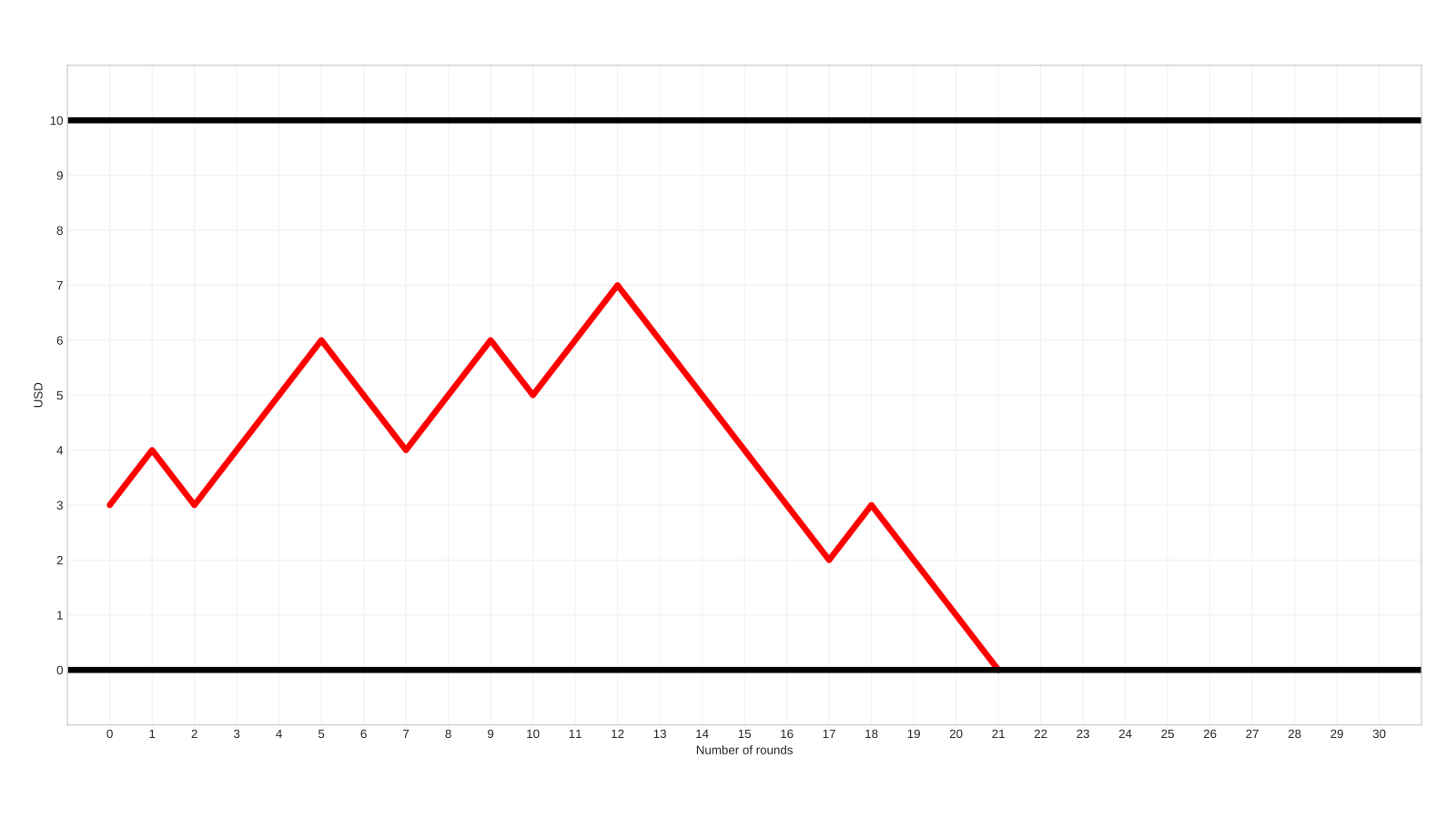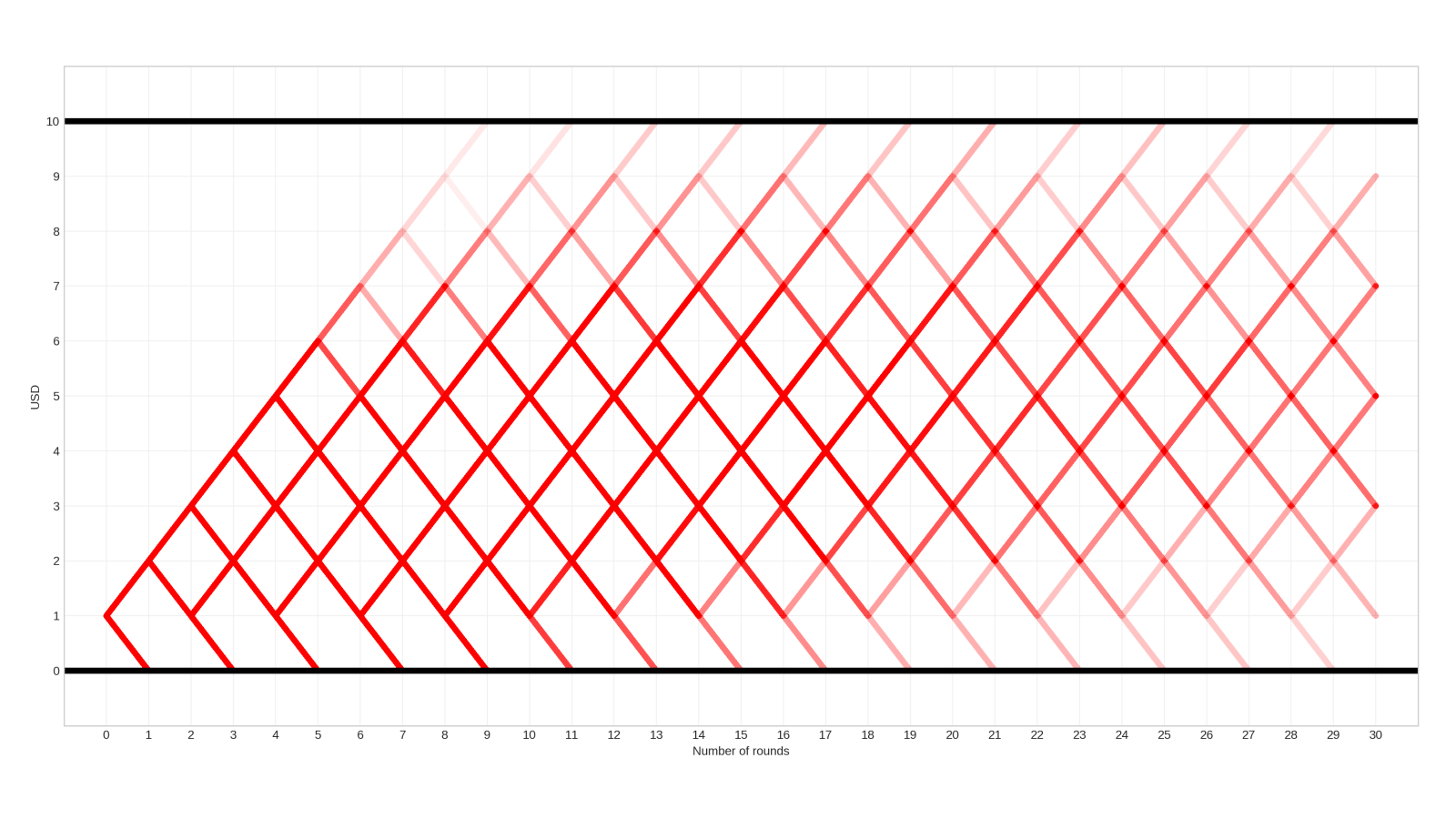# The gambler's ruin

One common wisdom about gambling is that the house always wins.

This is not just a catchphrase; there is mathematical evidence behind it. If you play against an opponent with much deeper pockets, your chances of winning approach zero.

To illustrate the problem above, consider a simple example: betting on coin tosses.

The dealer tosses a fair coin. If it lands on heads, you win $\1$. If tails, you lose $\1$. With each toss, the probability of winning and losing are the same:

$P(\text{winning a round}) = P(\text{losing a round}) = \frac{1}{2}.$

You have $\textstyle n$ dollars, while the casino has $\textstyle m$. In total, there are $N = n + m$ dollars on the table. You win when you reach $\textstyle N$ dollars. However, if you get to zero, you lose.

The question is simple: what is the probability of winning?

For instance, if you have $\3$ and the casino has $\7$, this is what one possible outcome looks like.($\textstyle x$ is the number of rounds, $\textstyle y$ is your balance.)

This problem is much simpler if we think outside the box and generalize the question. When we ask the probability of winning, conditioned on starting from n \$, a recursive relation and its boundary conditions are revealed. To put our idea into a mathematical form, consider the sequence

$p(n) := P(\text{winning }\N | \text{starting from }\n).$

It is easy to see that $p(0)=0$ and $p(N)=1$. What about the rest? For that, notice that when having $\n$, we either

• started from $\(n+1)$ and lost a round,
• or started from $\(n-1)$ and won a round.

Thus, we obtain the recursive relation

$p(n) = \frac{1}{2}p(n+1) + \frac{1}{2}p(n-1).$

Although this seems complicated, the solution is easier than you think! Solving this recursion, we obtain a simple formula: your probability of winning is proportional to the money you have.

$p(n) = \frac{n}{N}, \quad n = 0, 1, \dots, N.$

(If you are interested in working out the solution and the general case when the coin can be biased, check out this lecture from Harvard's Statistics course.)

If you are curious, I simulated $10 000$ trajectories and overlaid them on top of each other, with low opacity. (The paler a line is, the fewer trajectories go through it.) You can see here that starting from $\1$, losing is much more frequent than winning.Even though the game is fair (since winning or losing a round is equally probable), deeper pockets are advantageous. In real casinos, your account is tiny compared to theirs. Thus, in the long run, you are likely to lose.

Thus, the house (almost) always wins.

The truth is, casino games are never balanced. They are designed with probabilities that favor the house, so your chances are even worse than this simple mathematical model shows.

TL;DR: you don't stand a chance against a casino.